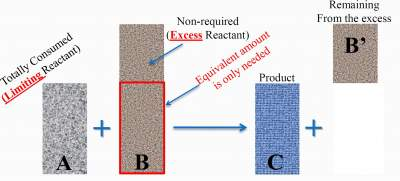# Limiting Reactant

## Introduction

### Example

2H2 + O2 → 2H2O
Each 2 moles of 2H2 need ONE mole of O2 to form 2 moles of H2 O
Assune we have 10 moles of H2, then we can ask the following:
How many moles of O2 are needed?

Solution:
5 moles

If we have only 10 mol of H2, 4 moles of O2.
Only 8 moles of H2 will be used leaving
2 unreacted(excess) H2 moles.
Meaning that the amount of O2 is limited of H2 needed
→ O2 is called "Limiting Reactant"
→ H2 is called "Excess Reactant"

Hence, at the end of the reaction:
• The limiting reactant will be completely consumed,
• Part of the excess reactant will remain after reaction

Important Remark:
In a "limiting reactant case", a limiting reactant is used to calculate the number of moles of the product since the limiting reactant is totally consumed in the reaction.

Typically, In a "limiting reactant case", the main task is to find the limiting reactant, then the excess reactant.
Sometimes, finding the excess reactant is easier and will lead to the limiting reactant.

### Example

For the reaction: A + B → C + B' (See illustration below):### Exercise

Exercise on Finding the Limiting Reactant

Check your answers here: Solution to the Exercise on Finding the Limiting Reactant

Important Remarks:
• Finding the "right" limiting reactant is crucial in these problems, because it is used to calculate the # mol of products to be formed.
• If number of mol of both reactants are given, then finding the "right" limiting reactant is straightforward.
• If number of grams of both reactants are given, then converting these numbers to mol is essential to find the "right" limiting reactant.
• If either number of mol or number of grams of both reactants are given, then it is a "limiting reactant problem", even if finding the "right" limiting is not asked for.
In fact, in these cases, finding the "right" limiting reactant is a must to continue with the problem.